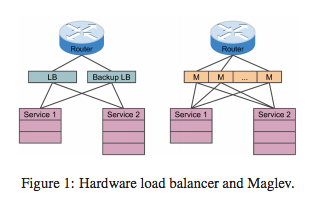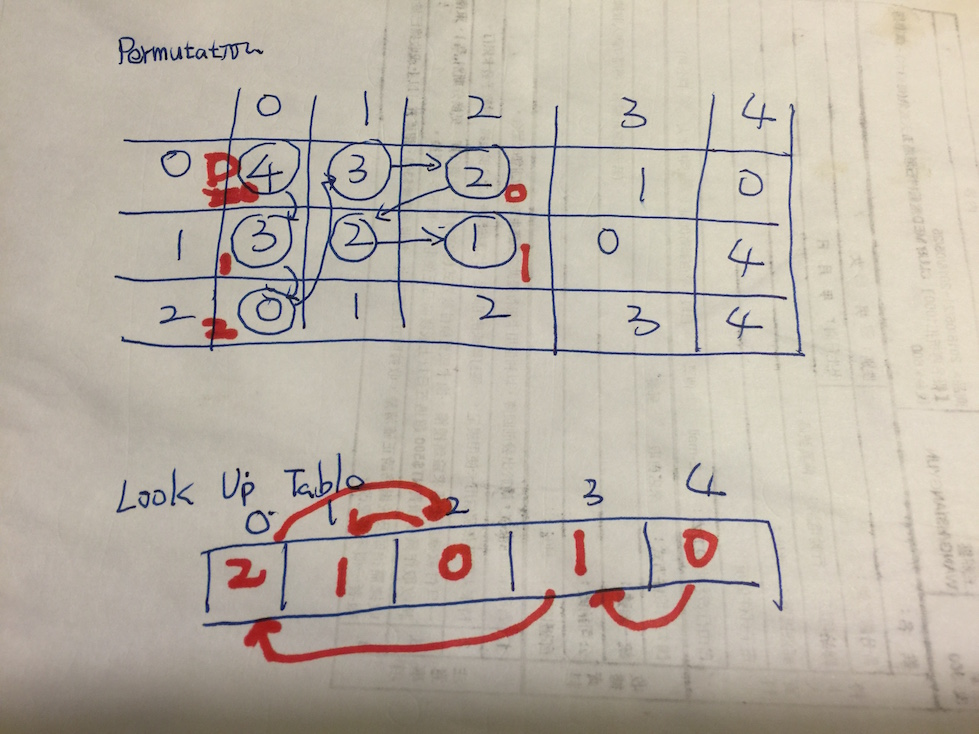## 前言 （為何想讀這一篇論文)

• 介紹 Maglev 的特性與其改善的部分
• 回顧 Consistent Hashing
• 介紹 Maglev Hashing

## 導讀

### 什麼是 Maglev?

Maglev 是 Google 的軟體 Load Balancer ，不像是一般硬體的 Load Balancer ， 他可以運行在一般的 Linux 機器上面． Maglev 在 Google 內部已經運行了超過 六年 ( since 2008 ) ．一台 Maglev 可以處理 10Gbps 的小封包連結．

### Maglev 主要的功能與特色

Maglev 作為 Google 內部的高效能軟體 Load Balancer ，他有以下兩個主要功能：

• 新的 Consistent Hashing Algorithm 稱為 Maglev Hashing
• Connection Tracking

### 回過來講，那什麼是 Consistent Hashing ?• 來源 ip : 1.2.3.4 透過將來源 ip 做 Hashing 過後指向 server1
• 來源 ip : 1.2.3.5 透過將來源 ip 做 Hashing 過後指向 server2
• 來源 ip : 1.2.4.6 透過將來源 ip 做 Hashing 過後指向 server3• Minimal Disruption : 這邊指的就是如果有節點被刪除，應該要達到只有該節點影響到的部分要修改而已． 在 Consistent Hashing 裡面透過選取下一個的方式． 透過將索引排序後，直接選取下一個節點作為 Hashing 後的結果節點．簡單的範例如下:
• 來源 IP 位置 1.2.3.4 ，經過 Hashing 後得到位置 1024 (假設)
• 到表格 1024 查詢資料，發現 1024 的節點伺服器 server1 已經出現故障．
• 尋找 1024 最接近的下一個節點 （假設是 1028 ) 並且對應到 server2
• 分配 server2• Load Balancing : 也就是盡可能地讓每個節點都能運用到，不會有某些節點有過分運用的疑慮． 在 Consistent Hashing 裡面是使用 Virtual Node ．
• 簡單的說，也就是在加入節點的時候，會一併複製數個虛擬節點 (通常使用 節點#1, 節點#2 ...  命名方式．
• 透過多個虛擬節點散佈在各地，讓尋找的時候更容易平均的分配到不同的節點．

### 對於 Maglev 而言，原本的 Consistent Hashing 有哪些缺點（限制)？

• 需要更均勻地分配每隔節點位置： 由於 Google 的每個節點可能都是數百台的機器，由於來源資料龐大，根據舊的演算法可能需要相當大的 lookup table 才能負荷．
• 需要更減少 Disruption : 對於 Google 的需求，演算法需要容忍小量的 disruption ．

### 關於 Maglev Hashing Algorithm 的介紹

#### 主要概念: 新增 Preference List 概念

Preference List (偏好清單) 會分配給每一個節點，讓每一個節點去填上自己偏好的位址( Permutation )．直到整個表格是填滿的狀態．

#### 效能:

• 平均狀況: $O(M log M)$
• 最差狀況: $O(M^2)$

• $M$ 是表示 lookup table 的大小．
• $N$ 是表示 節點的個數．

#### 流程:

• 首先 Maglev Hashing 會先把所有的 Preference List 產生出來．
• 透過產生好的 Preference List 開始將節點一個個地加入並且產生出Lookup table

### 程式碼分析:

#### 計算 “排列表格” Permutation Table

//name is the list of backend.
func generatePopulation() {
//如果 []name 是空的就離開
if len(name) == 0 {
return
}

for i := 0; i < len(name); i++ {
bData := []byte(name[i])

//計算 offset 透過 Hash K1
offset := siphash.Hash(0xdeadbabe, 0, bData) % M
//計算 skip 透過 Hash K2
skip := (siphash.Hash(0xdeadbeef, 0, bData) % (M - 1)) + 1

iRow := make([]uint64, M)
var j uint64
for j = 0; j < m.m; j++ {
//排列組合的表格
iRow[j] = (offset + uint64(j)*skip) % M
}

permutation = append(permutation, iRow)
}
}



#### 產生查表表格(Lookup Table)

• entry: 代表表格中有沒有走過．架設 lookup table 大小為 7，就得 0 ~ 6 都走過一次． （不然為 -1)．而最後裡面的數值就是節點的索引．
• next: 代表排列表格的下一個位置．如果節點有三個，那麼排列表格就有三組．於是 next 大小也有三個，分別記錄每一個排列表格走到第幾個位置．

#### 範例資料

unc (m *Maglev) populate() {
if len(m.nodeList) == 0 {
return
}

var i, j uint64
next := make([]uint64, m.n)
entry := make([]int64, m.m)
for j = 0; j < m.m; j++ {
entry[j] = -1
}

var n uint64

for { //true
for i = 0; i < m.n; i++ {
c := m.permutation[i][next[i]]
for entry[c] >= 0 {
next[i] = next[i] + 1
c = m.permutation[i][next[i]]
}

entry[c] = int64(i)
next[i] = next[i] + 1
n++

if n == m.m {
m.lookup = entry
return
}
}

}

}



N = 3
M = 5

m.permutation  = [4, 3, 2, 1, 0]
m.permutation  = [3, 2, 1, 0, 4]
m.permutation  = [0, 1, 2, 3, 4]



• 將剛剛建立出的排列表格拿出來
• i=0 ，從第一個排列表格的第一個挑出數值 c1=4 ，那麼 entry = 0 (代表 lookup table 中的 entry 是指向節點 0
• i=1 ，從第二個排列表格的第一個挑出數值 c2=3 ，那麼 entry = 1
• i=2 ，從第三個排列表個的第一個挑出數值 c3=0 ，那麼 entry = 2
• 重跑 i 迴圈， i=0 ． 從第一個排列表格的第二個( index=1 )挑出數值 c4=3 ，由於 entry 走過了，往後走一個 （next +1) 走到 m.permutation=2， 於是 entry=0
• 依此類推，直到所有的 n == M ．此時，也會發現 entry[] 不再存在任何 -1## Maglev Hashing 跟 Consistent Hashing 的比較

• Consistent Hashing
• 準備工作:
• 將每個節點數值根據 Hashing key 加入 lookup table
• 製作出 Virtual Node 來達到平衡．
• 如何查詢:
• 將數值透過 Hash Key 對應到一個 lookup table 的索引 index
• 如果該 index 沒有節點，往下尋找最接近的節點
• Maglev Hashing
• 準備工作:
• 需要先建立一個排列表格
• 並請需要先 透過排列表格做出偏好清單．注意這時候所有 lookup table 每一個索引都有一個節點分配．
• 如何查詢:
• 數值透過 Hash Key 對應到一個 lookup table 的索引 index
• 由於準備工作，該 index 必定存在數值
• 傳回節點資料